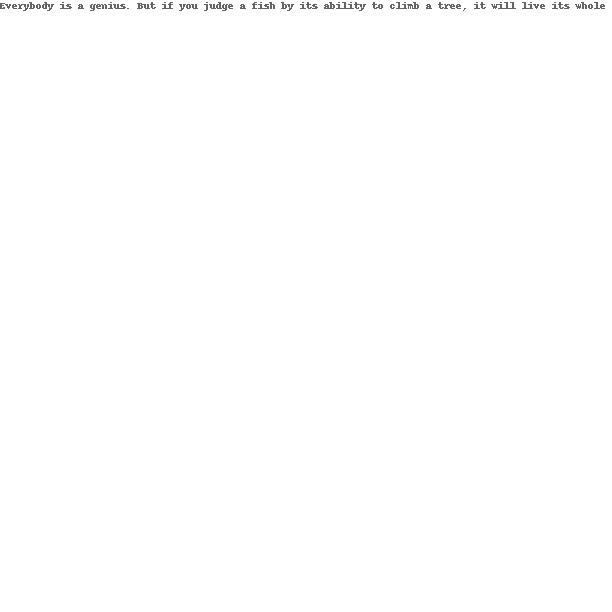# Instagram quotes generator using Python PIL

I am using Python’s Pillow library for image creation.You can even install it using pip.

`\$ pip install Pillow`

You can get the detailed installation instructions here.

Instagram’s default post size is 612px  612px so first let me create a 612px square white box.

```from PIL import Image, ImageDraw

#variables for image size
x1 = 612
y1 = 612

img = Image.new('RGB', (x1, y1), color = (255, 255, 255))
d = ImageDraw.Draw(img)

#save the image in the current directory
img.save('quote.png')```

Now lets put our quote inside this white box.

```from PIL import Image, ImageDraw

#variables for image size
x1 = 612
y1 = 612

#my quote
sentence = "Everybody is a genius. But if you judge a fish by its ability to climb a tree, it will live its whole life believing that it is stupid. -Albert Einstein"

img = Image.new('RGB', (x1, y1), color = (255, 255, 255))
d = ImageDraw.Draw(img)

#create the text at 0,0 location on the image
d.text((0,0), sentence ,align="center", fill=(100,100,100))

#save the image in the current directory
img.save('quote.png')```

Below is output image from the above code.Doesn’t look Instagram friendly. Lets make some changes to its font and see if it looks anywhere close to better. For this we will use ImageFont class.The quote isn’t fitting inside my box. I will need some line breaks between the words. And also need to center the quote in the middle of the box. The below code does the trick for me.

```from PIL import Image, ImageDraw, ImageFont

#variables for image size
x1 = 612
y1 = 612

#my quote
sentence = "Everybody is a genius. But if you judge a fish by its ability to climb a tree, it will live its whole life believing that it is stupid. -Albert Einstein"

#choose a font
fnt = ImageFont.truetype('/Users/muthukrishna/Library/Fonts/Cinzel-Regular.ttf', 30)

img = Image.new('RGB', (x1, y1), color = (255, 255, 255))
d = ImageDraw.Draw(img)

#find the average size of the letter
sum = 0
for letter in sentence:
sum += d.textsize(letter, font=fnt)

average_length_of_letter = sum/len(sentence)

#find the number of letters to be put on each line
number_of_letters_for_each_line = (x1/1.618)/average_length_of_letter
incrementer = 0
fresh_sentence = ''

for letter in sentence:
if(letter == '-'):
fresh_sentence += '\n\n' + letter
elif(incrementer < number_of_letters_for_each_line):
fresh_sentence += letter
else:
if(letter == ' '):
fresh_sentence += '\n'
incrementer = 0
else:
fresh_sentence += letter
incrementer+=1

print fresh_sentence

#render the text in the center of the box
dim = d.textsize(fresh_sentence, font=fnt)
x2 = dim
y2 = dim

qx = (x1/2 - x2/2)
qy = (y1/2-y2/2)

d.text((qx,qy), fresh_sentence ,align="center",  font=fnt, fill=(100,100,100))

img.save('quote.png')```

The output looks like thisAnother one on black background:Article Rating
Subscribe
Notify of1 Comment
Inline Feedbacks